Games
Problems
Go Pro!

# Number Theory

Lesson Plans > Mathematics > Number Theory

# Featured Lesson Plans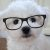142857 - Number theory, classroom projects, exploration, and activities surrounding an unusual number with mysterious properties.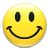Use Happy Numbers in your math classroom at either elementary or secondary level. A variety of educational uses.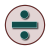Studying methods that can be used to determine whether a number is evenly divisible by other numbers is an important topic in elementary number theory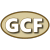Greatest Common Factor - easy method for finding it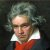Helping a child remember the difference between a prime number and a composite number - Which is which?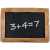Number theory topic: Modular Arithmetic, the arithmetic of remainders, and congrunecesThe factors of a natural number which are prime numbers are called the Prime Factors of that natural number.Comparison of Decimal (base 10) number system and Binary (base 2) number system, how to convert from one to the other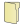# Full Directory Listing

Binary Number System : Conversion to and from Decimal System, Divisibility Rules for Prime Divisors, Mathematical Fun With Happy Numbers, Modular Arithmetic (Congruences) Of Elementary Number Theory, My Pet Number - 142857, Prime Factorization of Natural Numbers : Lucid ExplanationLike us on Facebook to get updates about new resources